The Generalized Triple Difference Lacunary Statistical on and#915;<sup>3</sup> Over P-Metric Spaces Defined by Musielak Orlicz Function | OMICS International
Journal of Physical Mathematics

# The Generalized Triple Difference Lacunary Statistical on Γ3 Over P-Metric Spaces Defined by Musielak Orlicz Function

Mishra LN1*, Deepmala2 and Subramanian N3

1Department of Mathematics, National Institute of Technology, Silchar – 788 010, India

2SQC & OR Unit, Indian Statistical Institute, Kolkata-700 108, India

3Department of Mathematics, Sastra University, Thanjavur-613 401, India

*Corresponding Author:
Mishra LN
Department of Mathematics
National Institute of Technology
India
Tel: +91 9838375431
E-mail: [email protected]

Received date: May 30, 2016; Accepted date: July 01, 2016; Published date: July 04, 2016

Citation: Mishra LN, Deepmala, Subramanian N (2016) The Generalized Triple Difference Lacunary Statistical on Γ3 Over P-Metric Spaces Defined by Musielak Orlicz Function. J Phys Math 7:183. doi:10.4172/2090-0902.1000183

Copyright: © 2016 Mishra LN, et al. This is an open-access article distributed under the terms of the Creative Commons Attribution License, which permits unrestricted use, distribution, and reproduction in any medium, provided the original author and source are credited.

Visit for more related articles at Journal of Physical Mathematics

#### Abstract

We introduce the generalized triple sequence spaces of entire difference lacunary statistical convergence and discuss general topological properties also inclusion theorems are with respect to a sequence of Musielak-Orlicz function.

#### Keywords

Analytic sequence; Triple sequences; Difference sequence; Γ3 space; Musielak-Orlicz function; Lacunary sequence; Statistical convergence

#### Introduction

A triple sequence (real or complex) can be defined as a function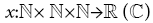where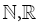and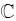denote the set of natural numbers, real numbers and complex numbers respectively. The different types of notions of triple sequence was introduced and investigated at the initial by Sahiner [1,2], Esi [3-5], Datta , Subramanian , Debnath  and many others.

A triple sequence x=(xmk) is said to be triple analytic if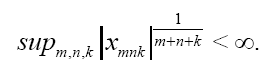The space of all triple analytic sequences are usually denoted by Λ3. A triple sequence x=(xmk) is called triple entire sequence if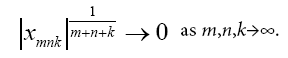The notion of difference sequence spaces (for single sequences) was introduced by Kizmaz  as follows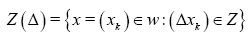For Z=c,c0 and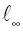where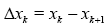for all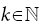The difference triple sequence space was introduced by Debnath et al. (see ) and is defined as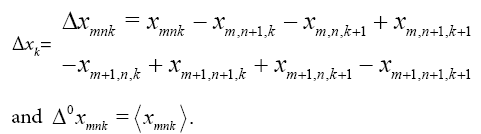#### Def﻿initions and Preliminaries

Throughout the article w33(Δ), Λ3(Δ) denote the spaces of all, triple entire difference sequence spaces and triple analytic difference sequence spaces respectively.

Subramanian introduced by a triple entire sequence spaces, triple analytic sequences spaces and triple gai sequence spaces . The triple sequence spaces of Γ3(Δ), Λ3(Δ) are defined as follows: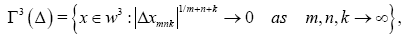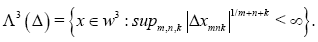1.Definition

An Orlicz function is a function M:[0,∞)→ [0,∞) which is continuous, non-decreasing and convex with M(0)=M(x)>0 forM(x)>0  and M(x)→∞ as x→∞. If convexity of Orlicz function M is replaced by M(x+y)≤ M(x)+ M(y) then this function is called modulus function. M:[0,∞)→ [0,∞)

Lindenstrauss and Tzafriri  used the idea of Orlicz function to construct Orlicz sequence space. A sequence g=(gmn) defined by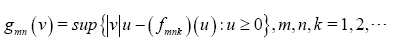is called the complementary function of a Musielak-Orlicz functionf. For a given Musielak-Orlicz function fthe Musielak-Orlicz sequence space tf is defined as follows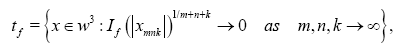Where If is a convex modular defined by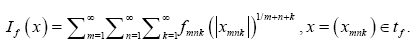We consider tf equipped with the Luxemburg metric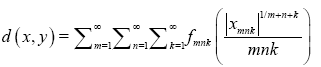is an exteneded real number.

2.Definition

Let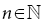X be a real vector space of dimension w where nm. A real valued function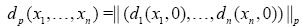on X satisfying the following four conditions:

(i)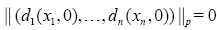if and and only if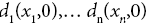are linearly dependent,

(ii)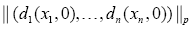is invariant under permutation,

(iii)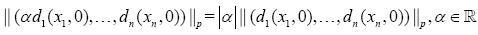(iv)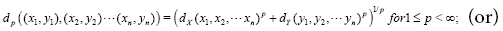(v)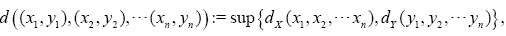,

For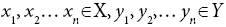is called the p product metric of the Cartesian product of n metric spaces .

3.Definition

Let X be a linear metric space. A function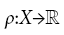is called paranorm, if

(1) ρ(x)≥0 for all xX;

(2) ρ(-x)= ρ(x) for all xX;

(3)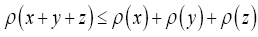, for all x,y,z∈X,

(4) If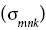is a sequence of scalars with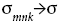as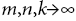and x=(xmk) is a sequence of vectors with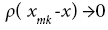asthen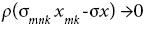as4.Definition

The triple sequence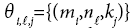is called triple lacunary if there exist three increasing sequences of integers such that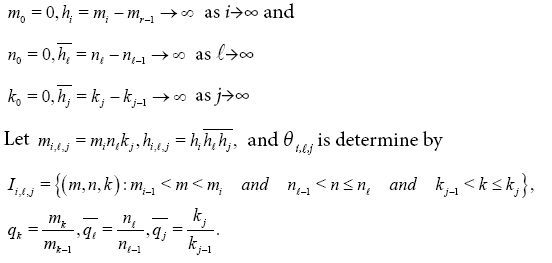#### Main Results

The notion of λ-triple entire and triple analytic sequences as follows: Let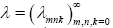be a strictly increasing sequences of positive real numbers tending to infinity, that is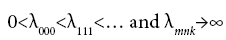and said that a sequence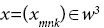is λ-convergent to 0, called a the λ-limit of x if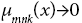aswhere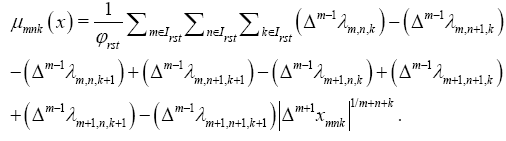The sequenceis λ-triple difference analytic if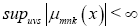. If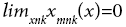in the ordinary sense of convergence, then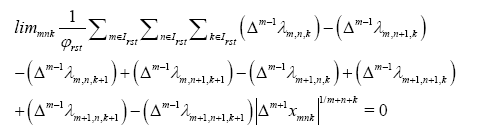This implies that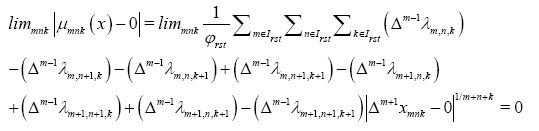which yields that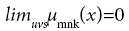and henceis λ-convergent to 0. Let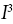- be an admissible ideal of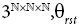be a triple difference lacunary sequence,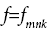be a Musielak-Orlicz function and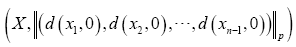be a p-metric space, q=(qmnk) be triple difference analytic sequence of strictly positive real numbers. By w3(p-X) we denote the space of all sequences defined over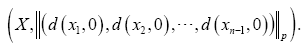In the present paper we define the following sequence spaces: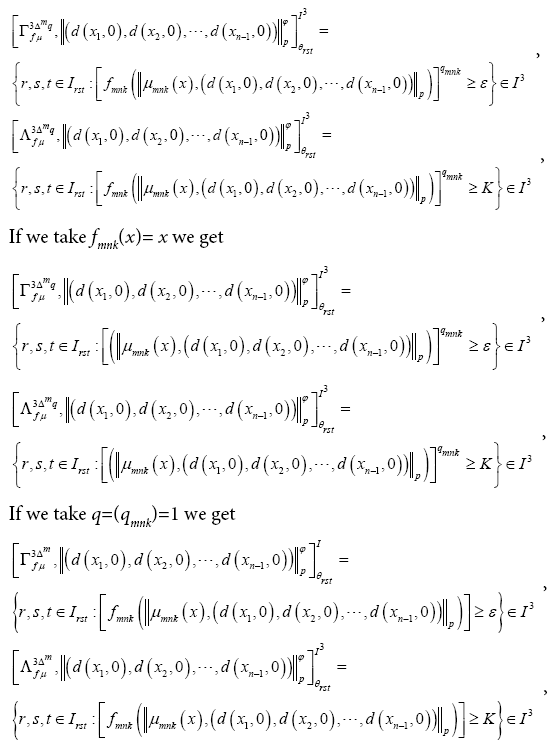In the present paper we plan to study some topological properties and inclusion relation between the above defined sequence spaces.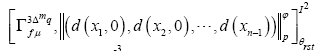and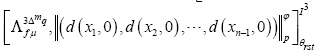which we shall discuss in this paper.

1.Theorem

Letbe a Musielak-Orlicz function, q=(qmnk) be a triple analytic difference sequence of strictly positive real numbers, the sequence spaces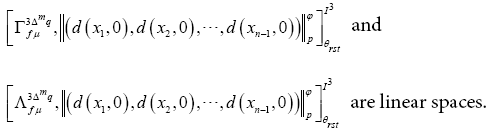Proof: It is routine verification. Therefore the proof is omitted.

2.Theorem

Letbe a Musielak-Orlicz function, q=(qmnk) be a triple analytic difference sequence of strictly positive real numbers, the sequence space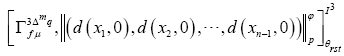is a paranormed space with respect to the paranorm defined by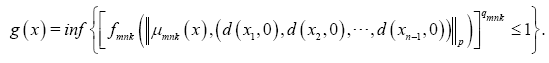Proof: Clearly g(x)≥0 for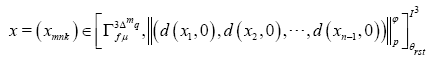Since fmnk(0)=0 we get g(0)=0

Conversely, suppose that g(x) then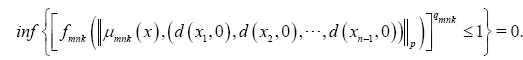Suppose that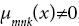. for each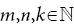Then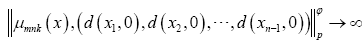. It follows that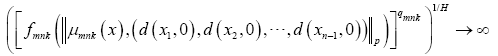which is a contradiction. Therefore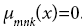. Let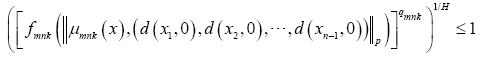and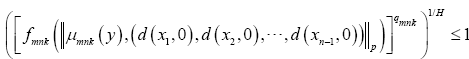Then by using Minkowski’s inequality, we have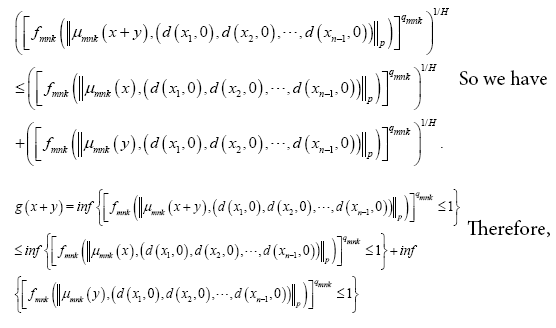g (x + y) ≤ g (x) + g ( y).

Finally, to prove that the scalar multiplication is continuous. Let λ be any complex number. By definition,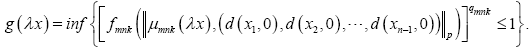Then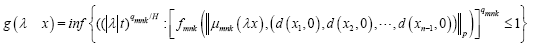where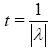. Since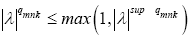, we have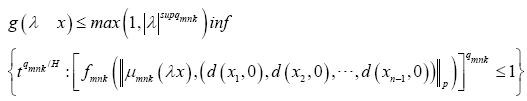This completes the proof.

3.Theorem

(i) If the Musielak Orlicz function (fmnk) satisfies Δ2- condition, then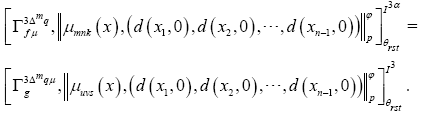(ii) If the Musielak Orlicz function (gmnk) satisfies Δ2- condition, then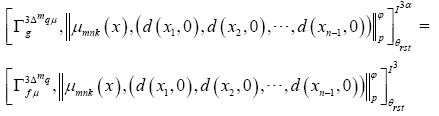Proof: Let the Musielak Orlicz function (fmk) satisfies Δ2-condition, we get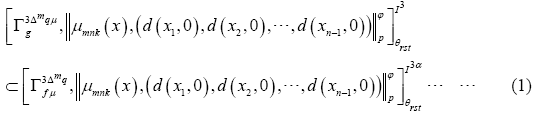To prove the inclusion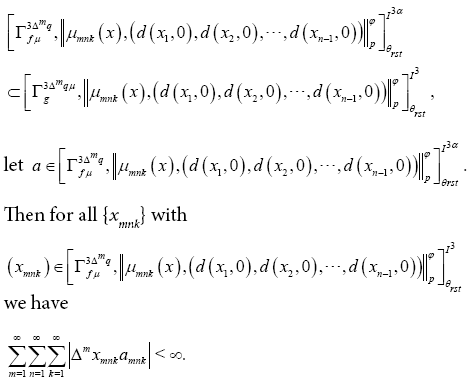Since the Musielak Orlicz function (fmk) satisfies condition, then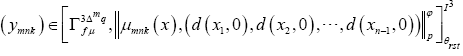, we get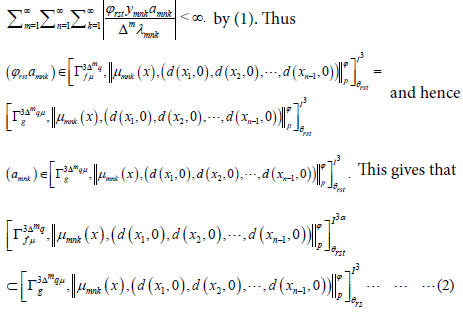we are granted with (1) and (2)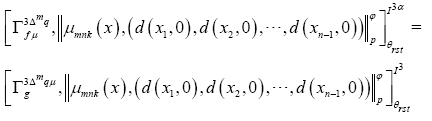(ii)Similarly,onecanprovethat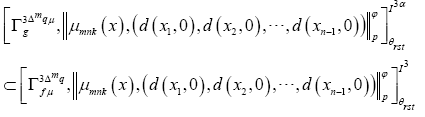if the Musielak Orlicz function (gmk) satisfies Δ2-condition.

1.Proposition

The sequence space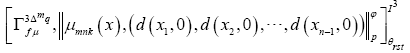is not solid Proof: The result follows from the following example.

Example: Consider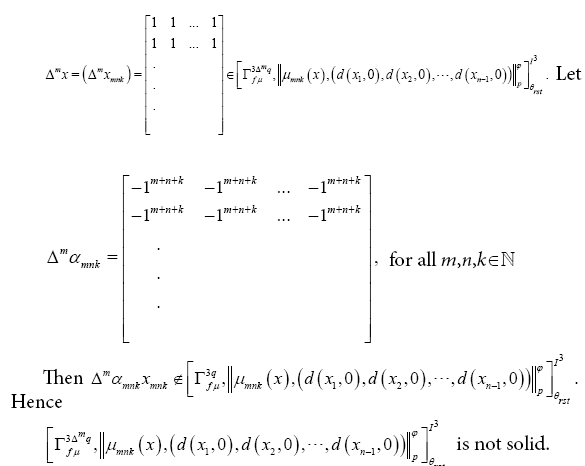2.Proposition

The sequence spaceis not monotone.

Proof: The proof follows from Proposition 3.4.

3.Proposition

The sequence space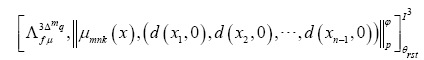is not solid.

4.Proposition

The sequence spaceis not monotone.

#### Conclusion

Through this paper we studied some topological properties and inclusion relation with respect to a sequence of Musielak-Orlicz function.

#### References

Select your language of interest to view the total content in your interested language

### Article Usage

• Total views: 10099
• [From(publication date):
September-2016 - Dec 15, 2019]
• Breakdown by view type
• HTML page views : 9904Can't read the image? click here to refresh